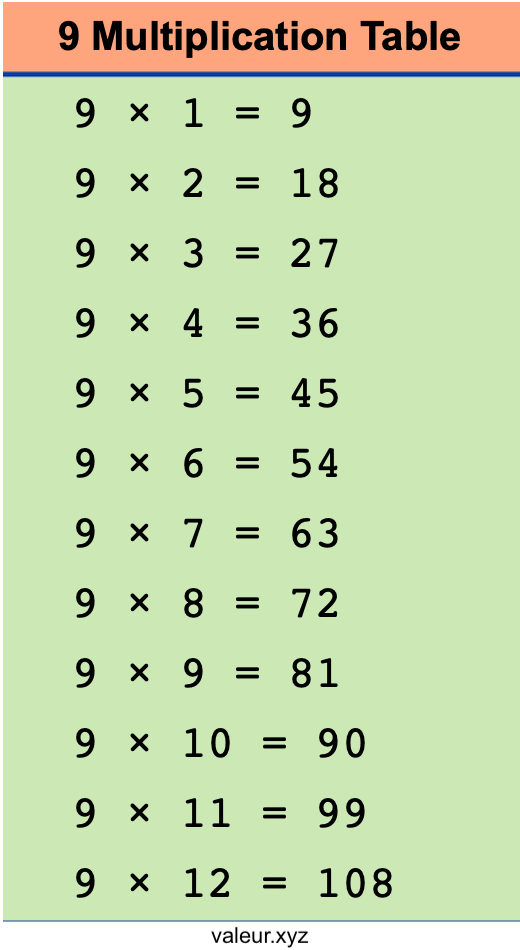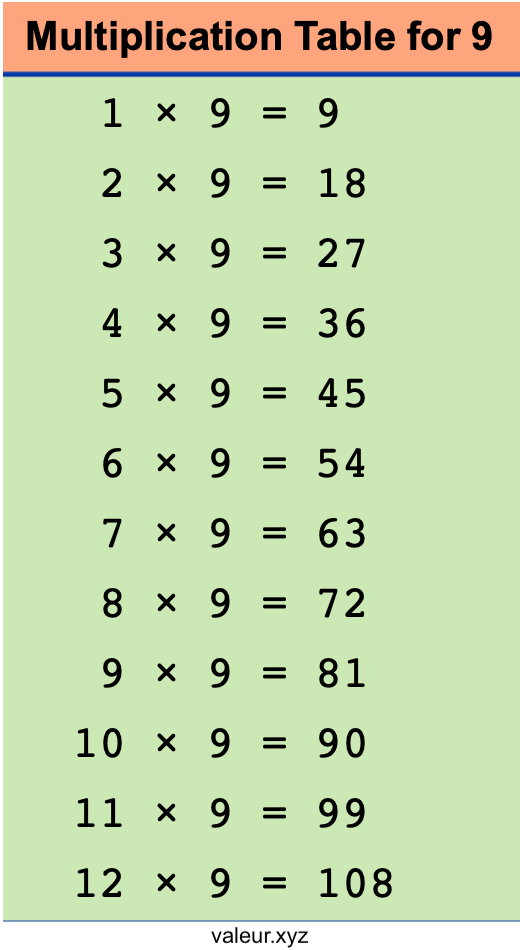9 Multiplication Table

We created a free printable multiplication table for 9 for you, so you can print it out and learn or teach someone the 9 times table.

As you can see below, we actually created two multiplication tables for 9. We made two versions of the 9 times table to illustrate that 1 times 9 is the same as 9 times 1.

Save and print the table below that you think will work best for you or your students to learn the multiplication table for 9.Multiplication Tables
Print out all of our multiplication tables! Submit the multiplication table of your choice below.

10 Multiplication Table
Here is the next multiplication table in our database that you can print out for free.